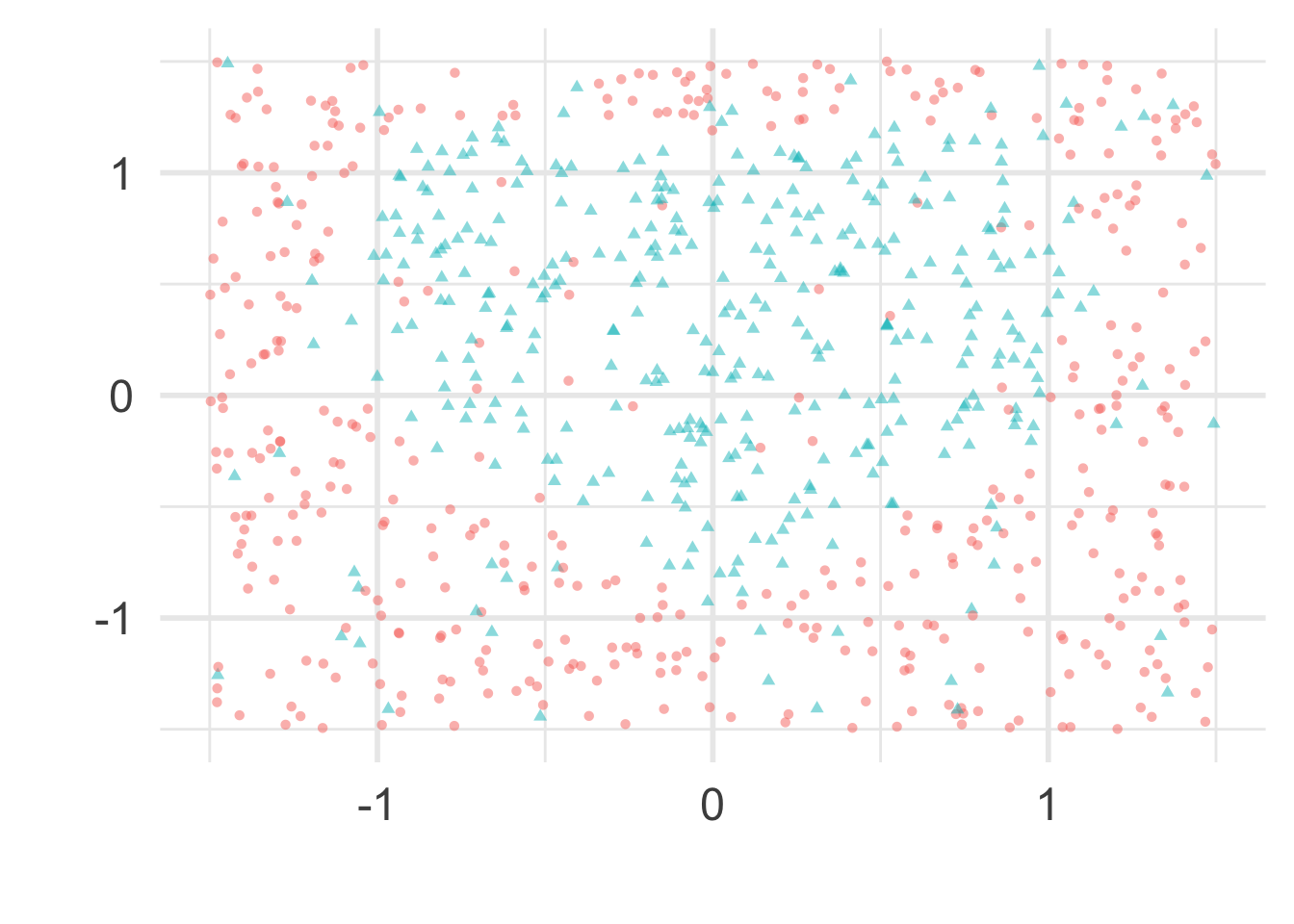## 2d non-linear classification data

``````# sample size
n <- 800
# function with zero set that
# defines the perfect (Bayes) decision boundary
true_boundary_function <- function(x1, x2) {
# experiment with changing this
thing <- x1^2*x2^3
(x1^2 + x2^2 - 1)^3 - thing
}
train <- data.frame(
# change the distribution
# or scale as needed
x1 = 1.5*(1 - 2*runif(n)),
x2 = 1.5*(1 - 2*runif(n))
) %>%
mutate(
# labels if classes were perfectly separated
separable = true_boundary_function(x1,x2) > 0,
# labels if classes are "noisy"
y = factor(rbinom(n, 1, 9/10 - (8/10) * as.numeric(separable)))
)``````

### Plot the data

``````train_plot <-
ggplot(train, aes(x1, x2)) +
geom_point(aes(shape = y, color = y),
alpha = .5, show.legend = FALSE) +
xlab("") + ylab("")

train_plot``````### Plot the Bayes decision boundary

``````decision_surface <-
data_grid(train,
x1 = seq_range(x1, 300, expand = .05),
x2 = seq_range(x2, 300, expand = .05)) %>%
mutate(z = true_boundary_function(x1, x2))

boundary_plot <-
train_plot +
geom_contour(
data = decision_surface,
aes(x1, x2, z=z),
bins = 2,
size = 1,
color = "black",
alpha = .5)

boundary_plot``````